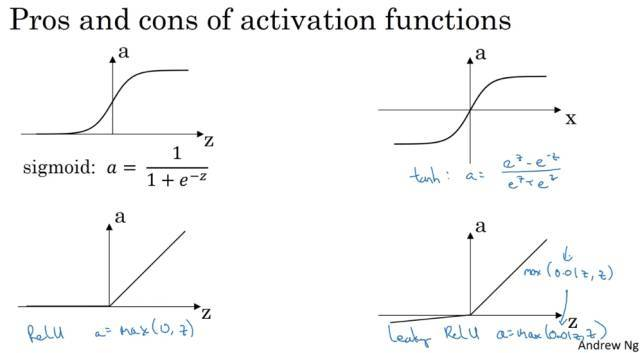# 3.1+3.2 神经网络概览+神经网络表示“隐藏层”这个名字的含义是：在训练集中，这些中间节点的真正数值我们是不知道的，在训练集中你看不到他们的数值（训练集只有输入值和输出值），“隐藏”就是想表示无法在训练集中看到。也就是不知道中间过程。只知道输入和输出。

# 3.3 计算神经网络的输出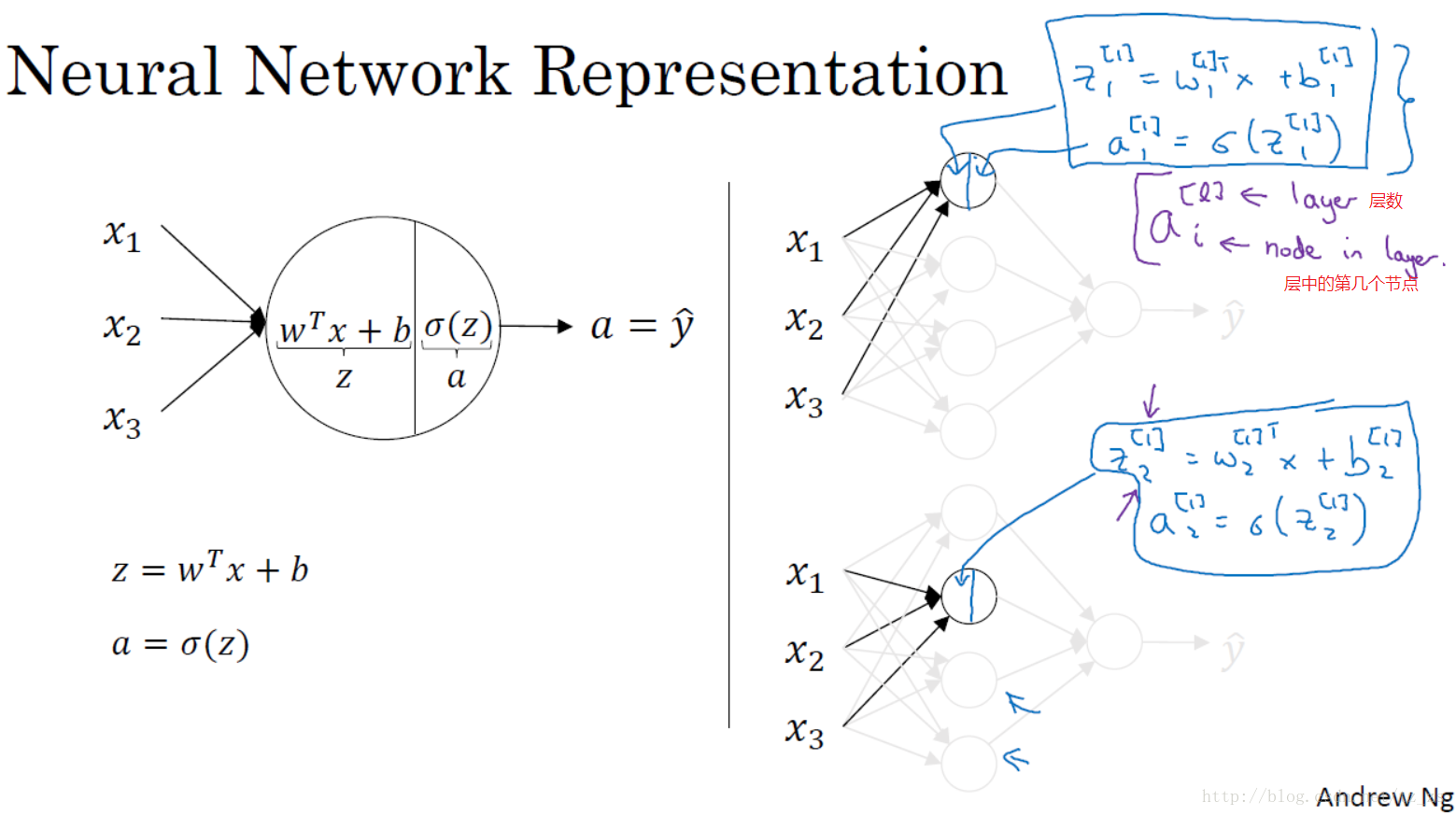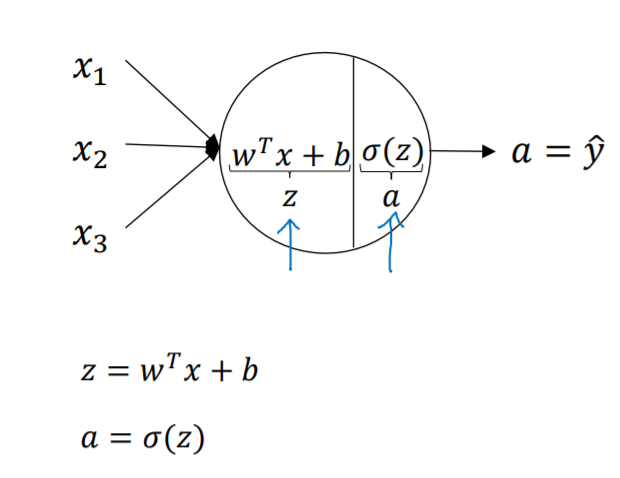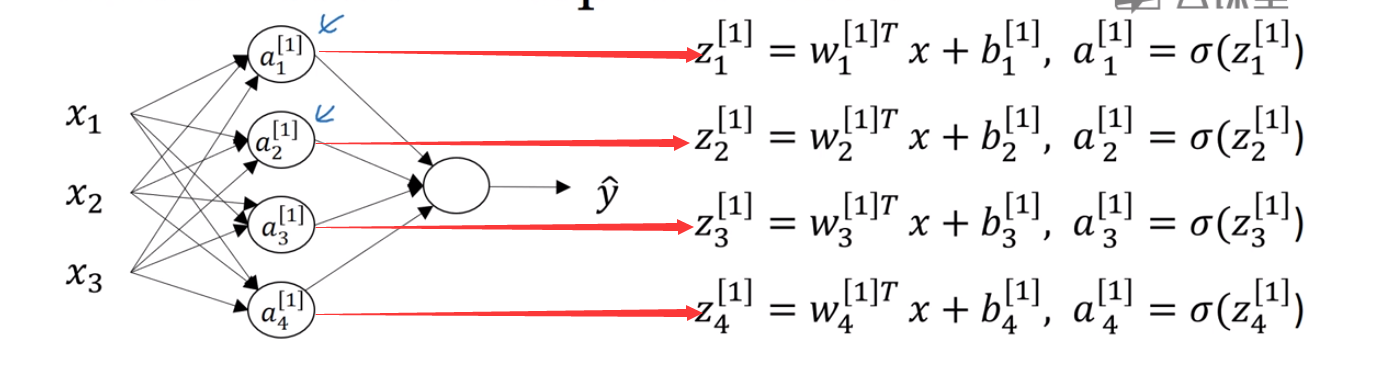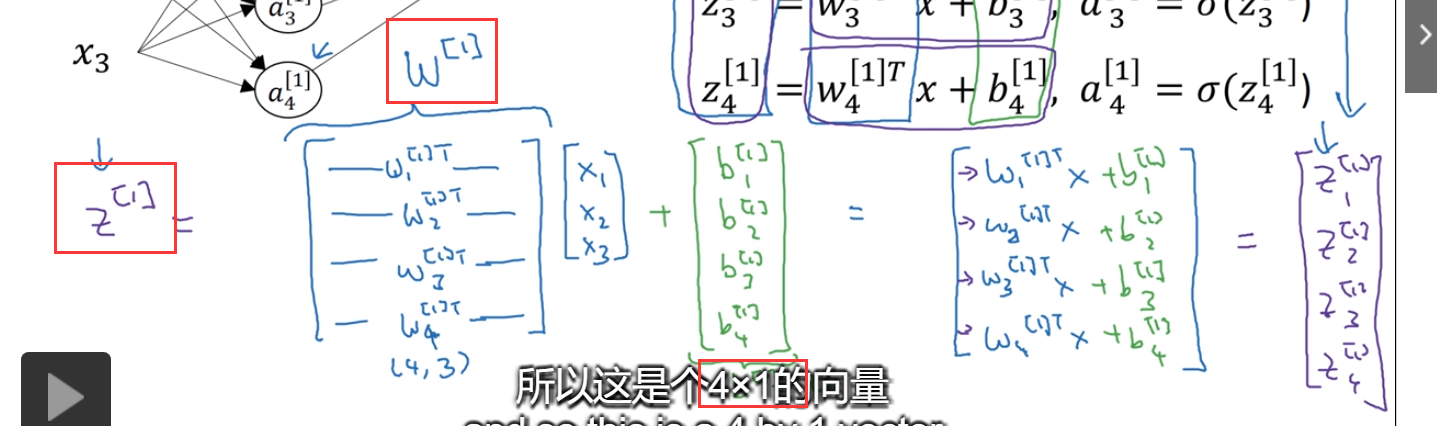# 3.4+3.5 多样例下的向量化+验证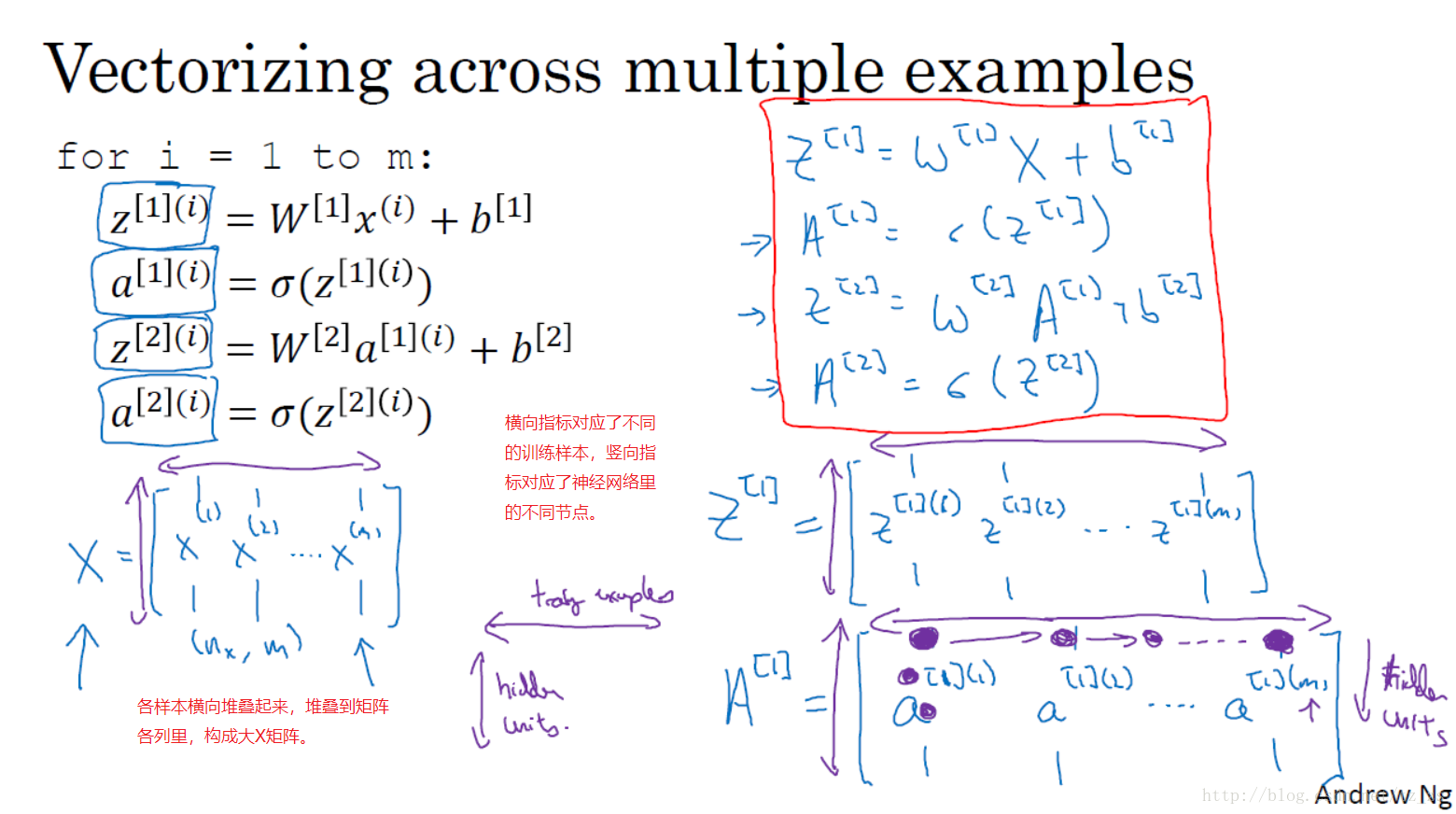# 3.6 激活函数

## Sigmoid函数

$σ(z)=\frac{1}{1+e^{−z}}$

## 双曲正切函数

$tanh(z)=\frac{e^z−e^{−z}}{e^z+e^{−z}}$

## ReLu函数(The Rectified Linear Unit)

（因为大家都在用）

1.梯度不饱和。$x>0$ 时梯度为1。因此在反向传播过程中，减轻了梯度弥散的问题，神经网络前几层的参数也可以很快的更新。

2.计算速度快。正向传播过程中，sigmoid和tanh函数计算激活值时需要计算指数，而Relu函数仅需要设置阈值。如果$x<0,f(x)=0$，如果$x>0,f(x)=x$。加快了正向传播的计算速度。

## Leaky Relu